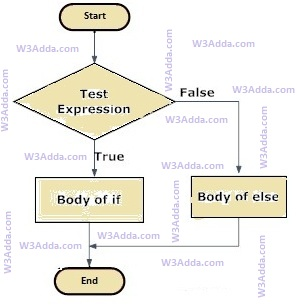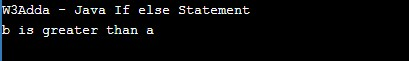# Java if else statement

In this tutorial you will learn about the Java if else statement and its application with practical example.

## Java if-else statement

In Java, when we want to execute a block of code when if the condition is true and another block of code when if the condition is false, In such a case we use the if-else statement.

## Java If…else Statement Flow DiagramSyntax:-

Here, Condition is a Boolean expression that results in either True or False, if it results in True then statements inside if body are executed, if it results in False then statements inside else body are executed.

Example:-

Output:-In this tutorial we have learn about the Java if else statement and its application with practical example. I hope you will like this tutorial.